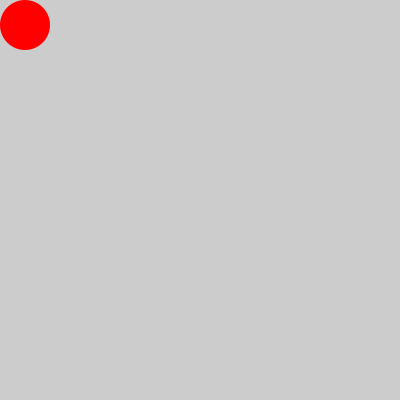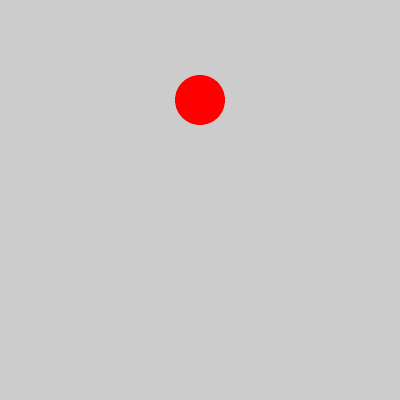# Creating GIFs in generativepy

Martin McBride, 2020-10-02
Tags generativepy tutorial gif
Categories generativepy generativepy tutorial

generativepy has moved to the pythoninformer.com website, and this page might not be up to date. Please visit the new location.

generativepy makes it very easy to create animated GIFs. This can be done with a simple script, and usually results in a fairly well optimised GIf file.

The `gif` module makes use of the gifsicle application. This can be downloaded for free, and must be installed on your system in order for the `gif` module to work.

## GIF images

GIF (Graphics Interchange Format) is a bitmap image format that was developed in the 1980s for transferring images over networks. It uses LZW compression (similar to the method used by ZIP files), which was more efficient than most other formats at the time.

Due to its efficiency, it was very popular in the early days of the web, when most people (especially home users) had quite slow connections, so efficient image compression was very important. However, these days it has largely been replaced by PNG and JPEG formats, which offer better compression and better image quality.

One feature of GIF is that it allowed several images to be stored in the same file, which could them be displayed sequentially to provide an animated effect. No other image format offers this animation feature, and certainly not in a format that is so widely supported.

## How GIF animation works

An animated GIF stores several images in the same file. It also specifies a time delay between frames, which is effectively the frame rate of the animation. A GIF viewer will automatically display the images one after another, with the specified delay, which creates an animated image.

GIF animation works in a similar way to video formats, which also store multiple frames in one file. However, video formats typically use far more sophisticated compression techniques, which achieve a much smaller file size. Animated GIF is not a video format, if you tried to store a 5 minute, high quality video as a GIF file, it would a huge file. You should be aware of the limitations:

• A GIF file will often contain less than a hundred frames.
• The frame will typically be between about 10 and 25 frames per second.
• This means that GIFs are almost always short in duration, typically a few seconds.
• In addition, each frame of a GIF can only contain 256 different colours.

The lack of colours and low frame rate means that video clips, for example from a movie, are quite poor quality. However, GIFs are great for animated vector graphics, and generativepy is a great way to create mathematical animations.

## Creating a simple GIF

Here is a simple GIF image of a red circle moving across the image:Here is the code that creates the GIF:

```from generativepy.drawing import setup
from generativepy.color import Color
from generativepy.geometry import Circle
from generativepy.gif import save_animated_gif
from generativepy.movie import make_frames

FRATE = 25
FRAMES = 100

def draw(ctx, width, height, frame_no, frame_count):

setup(ctx, width, height, background=Color(0.8))

pos = (25 + frame_no*3.5, 25 + frame_no*2)
.fill(Color('red'))

frames = make_frames(draw, 400, 400, FRAMES)
save_animated_gif('simple-gif.gif', frames, 1/FRATE)
```

In this code, we first define our `draw` function, that does the actual drawing (described later). The final two lines of code create the GIF:

```frames = make_frames(draw, 400, 400, FRAMES)
save_animated_gif('simple-gif.gif', frames, 1/FRATE)
```

`make_frames` is a function from the `generativepy.movie` module. It accepts our `draw` function, the image size (400 by 400 pixels in this case), and the total number of frames (100 in this case).

What `make_frames` actually does is to call `draw` 100 times, to generate a sequence of 100 frames.

We then pass that sequence of frames into `save_animated_gif`, which creates an animated GIF file simple-gif.gif. The function accepts a frame time parameter than controls the frame rate. Our required frame rate, `FRATE`, is 25 frames per seconds, so we pass in a frame time `1/FRATE` (ie 0.04 seconds per frame).

### The draw function

```def draw(ctx, width, height, frame_no, frame_count):

setup(ctx, width, height, background=Color(0.8))

pos = (25 + frame_no*3.5, 25 + frame_no*2)
.fill(Color('red'))
```

The `draw` function is called once per frame (ie 100 times in our case). It takes the following parameters:

• `ctx` - the Pycairo context we will be drawing on.
• `width`, `height` - the image width and height in pixels.
• `frame_no` - the number of the current frame. This will be 0 for the first frame, 1 for the second frame, and so on, up to 99 for the final frame. We use the frame number inside the `draw` function to vary the image for each frame, to create the animation.
• `frame_count` is the total number of frames (100 in this case).

The content of our `draw` function is quite simple:

• We call `setup` to set the background colour to light grey.
• We calculate the position `pos` of the circle, based on the `frame_no`. The first frame has a position of (25, 25), and as `frame_no` increments this position gradually changes towards (375, 225). On each frame, the circle is drawn at a slightly different location, creating a smooth movement.
• `Circle` draws a red circle, diameter 25 pixels, centred at `pos`.

## Tweening

Tweening allows you to specify parameters such as size, position, colour etc for certain frames of the animation. These are called key frames. The parameter values for the other frames are calculated automatically by interpolation. This allows you, for example, to specify the start and end positions of an object, and have it move smoothly between those positions.

generativepy provides a simple tweening library, which we will use here to move our circle and gradually change its colour. Here is the code:

```from generativepy.drawing import setup
from generativepy.color import Color
from generativepy.geometry import Circle
from generativepy.gif import save_animated_gif
from generativepy.movie import make_frames
from generativepy.tween import Tween, TweenVector

FRATE = 25
FRAMES = 200

x = Tween(200).to(300, 50).to(100, 100).to(200, 50)
y = Tween(100).to(300, 100).to(100, 100)
c = TweenVector((1, 0, 0)).to((0, 1, 0), 100).to((0, 0, 1), 100)

def draw(ctx, width, height, frame_no, frame_count):

setup(ctx, width, height, background=Color(0.8))

pos = (x[frame_no], y[frame_no])
.fill(Color(*c[frame_no]))

frames = make_frames(draw, 400, 400, FRAMES)
save_animated_gif('simple-gif2.gif', frames, 1/FRATE)
```

The `x` and `y` positions of the circle are controlled by the following tweens:

```x = Tween(200).to(300, 50).to(100, 100).to(200, 50)
y = Tween(100).to(300, 100).to(100, 100)
```

This means that:

• the `x` value starts at 200, moves to 300 over the first 50 frames, moves to 100 over the next 100 frames, then moves back to 200 over the final 50 frames.* the `y` value starts at 100, moves to 300 over the first 100 frames, then moves back to 100 over the final 100 frames.

Within the `draw` function, we can obtain the position for a particular frame like this:

```    pos = (x[frame_no], y[frame_no])
```

This calculates the position for any `frame_no` between 0 and 199. The `x` and `y` values defined by the tween cause the position to describe a diamond shape over 200 frames.

We also calculate a colour value using `TweenVector`. This is similar to `Tween` but it uses a vector value. We are using a 3-vector that represents an RGB colour.

```c = TweenVector((1, 0, 0)).to((0, 1, 0), 100).to((0, 0, 1), 100)
```

Here the colour starts at (1, 0, 0) which is red, gradually changes to (0, 1, 0) which is green, and then gradually changes again to (0, 0, 1) which is blue. We convert the vector quantity into a colour in the `draw` function, like this:

```Color(*c[frame_no])
```

In this code, `c[frame_no]` gives the colour values for a particular frame. For example, `c` is halfway between the first value (1, 0, 0) and the second value (0, 1, 0), which gives a value of (0.5, 0.5, 0). We unpack this value and pass it into the `Color` constructor, which takes 3 parameters for the r, g, and b values.

Here is the final animation. The circles moves around a diamond shape, as its colour changes from red to green to blue: